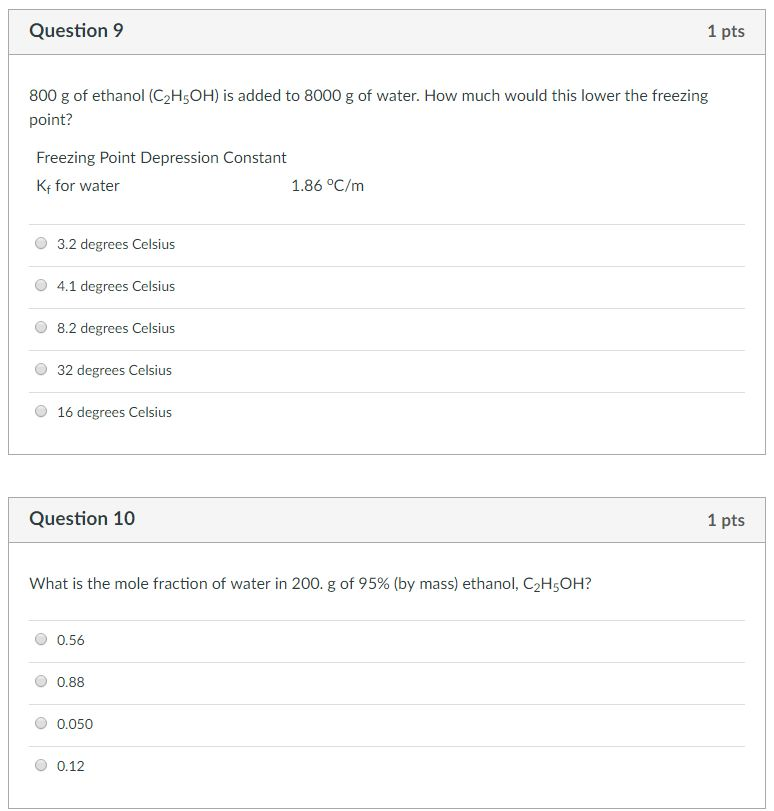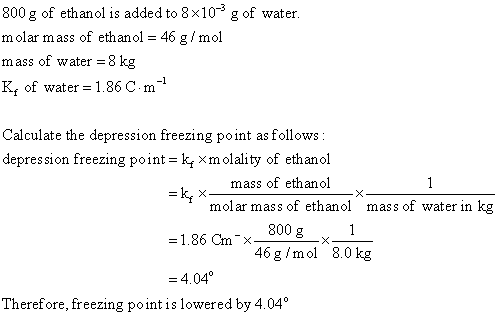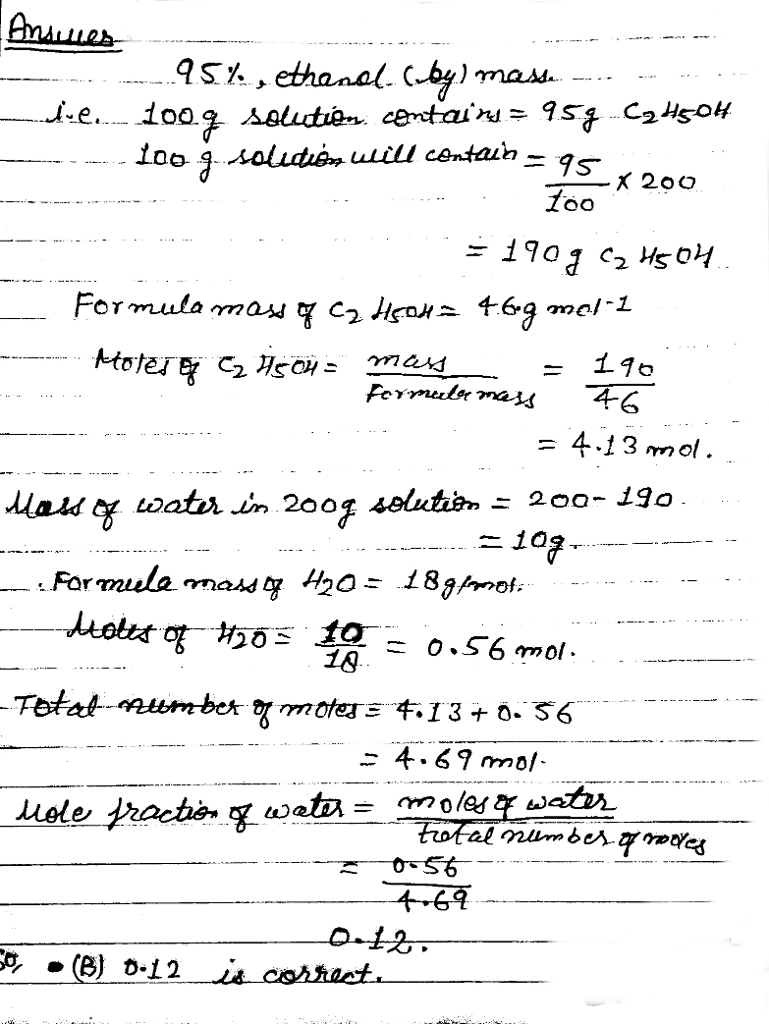# Question & Answer: 800 g of ethanol (C_2H_5OH) is added to 8000 g of water. How much would this lower…..800 g of ethanol (C_2H_5OH) is added to 8000 g of water. How much would this lower the freezing point? Freezing Point Depression Constant K_f for water 1.86 degree C/m 3.2 degrees Celsius 4.1 degrees Celsius 8.2 degrees Celsius 32 degrees Celsius 16 degrees Celsius What is the mole fraction of water in 200, g of 95% (by mass) ethanol, C_2H_5OH? 0.56 0.88 0.050 0.12